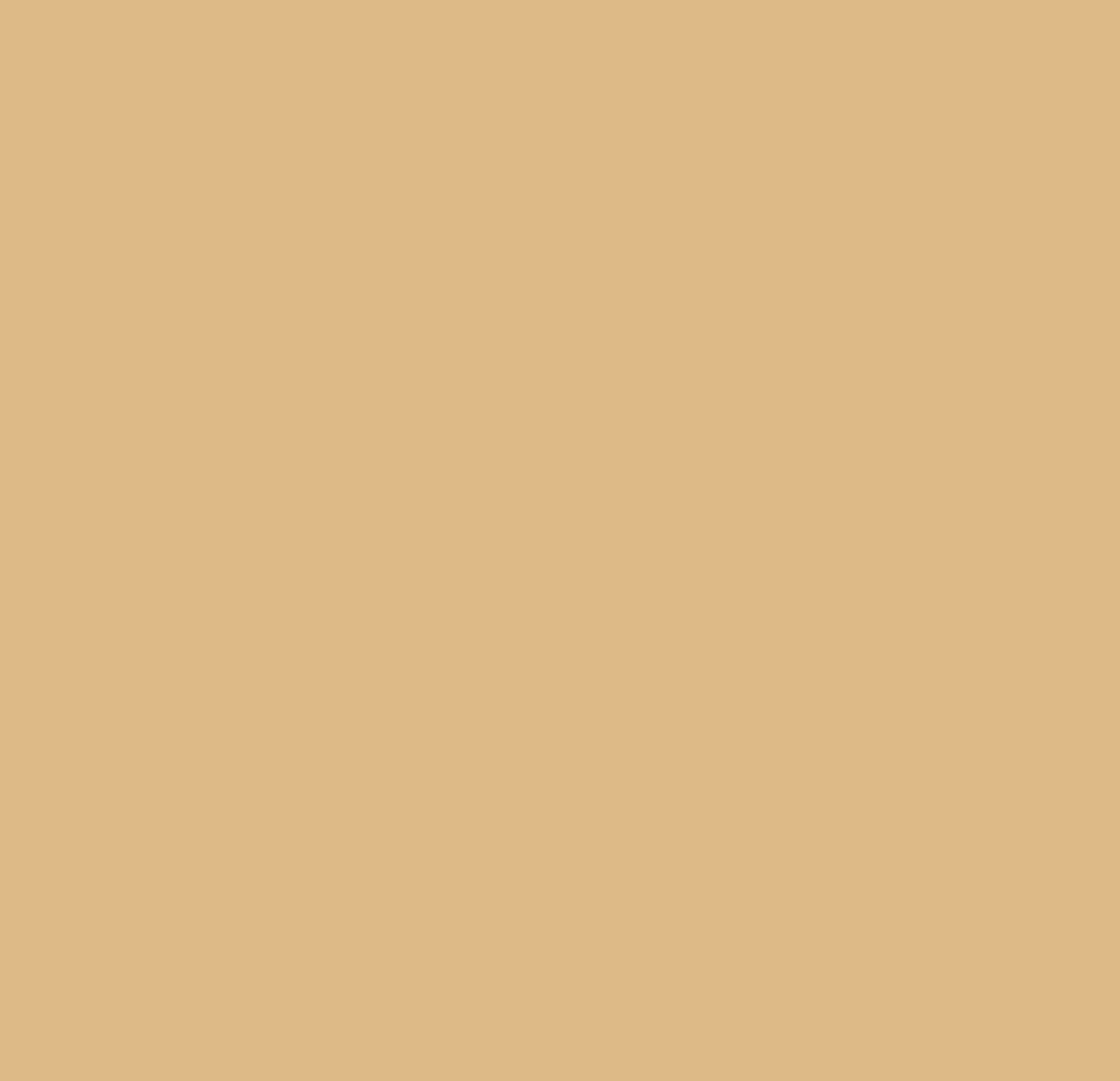ROOT   Reference Guide
Searching...
No Matches
glparametric.C File Reference

## Detailed Description

Show rendering of parametric surfaces.

A parametric surface is defined by three functions: S(u, v) : {x(u, v), y(u, v), z(u, v)}. To create parametric surface and draw it one has to:

1. Create canvas, which support OpenGL drawing (two ways):
• Call gStyle->SetCanvasPreferGL(kTRUE)
• Or create canvas with name, wich contains "gl".
2. create TGLParametricEquation object.
"some FORMULA here - x(u, v)",
"some FORMULA here - y(u, v)",
"some FORMULA here - z(u, v)",
uMin, uMax, vMin, vMax);
A parametric surface is a surface defined by a parametric equation, involving two parameters (u,...
where FORMULA is the same string (mathematical expression), as in TF2, but you should use 'u' (or 'U') instead of 'x' and 'v' (or 'V') instead of 'y'.
3. Call equation->Draw(); Parametric surfaces support 21 color "schemes", you can change the color:
• place mouse cursor above surface (surface is selected in pad)
• press 's' or 'S'.void glparametric()
{
TCanvas *c = new TCanvas("canvas","Parametric surfaces with gl", 100, 10,
700, 700);
c->SetFillColor(42);
c->Divide(2, 2);
c->cd(1);
"1.2 ^ u * (1 + cos(v)) * cos(u)",
"1.2 ^ u * (1 + cos(v)) * sin(u)",
"1.2 ^ u * sin(v) - 1.5 * 1.2 ^ u",
0., 6 * TMath::Pi(), 0., TMath::TwoPi());
p1->Draw();
c->cd(2);
"cos(u) * (4 + 3.8 * cos(v)) ",
"sin(u) * (4 + 3.8 * cos(v))",
"(cos(v) + sin(v) - 1) * (1 + sin(v)) * log(1 - pi * v / 10) + 7.5 * sin(v)",
p2->Draw();
c->cd(3);
"(abs(u) - 1) ^ 2 * cos(v)",
"(abs(u) - 1) ^ 2 * sin(v)",
"u",
-1., 1., 0, TMath::TwoPi());
p3->Draw();
c->cd(4);
TGLParametricEquation *p4 = new TGLParametricEquation("Trangluoid trefoil",
"2 * sin(3 * u) / (2 + cos(v))",
"2 * (sin(u) + 2 * sin(2 * u)) / (2 + cos(v + 2 * pi / 3))",
"(cos(u) - 2 * cos(2 * u)) * (2 + cos(v)) * (2 + cos(v + 2 * pi / 3)) / 4",
p4->Draw();
}
#define c(i)
Definition RSha256.hxx:101
const Bool_t kTRUE
Definition RtypesCore.h:91
R__EXTERN TStyle * gStyle
Definition TStyle.h:412
The Canvas class.
Definition TCanvas.h:23
virtual void Draw(Option_t *option="")
Default Draw method for all objects.
Definition TObject.cxx:197
void SetFrameFillColor(Color_t color=1)
Definition TStyle.h:355
void SetCanvasPreferGL(Bool_t prefer=kTRUE)
Definition TStyle.h:325
constexpr Double_t Pi()
Definition TMath.h:37
constexpr Double_t TwoPi()
Definition TMath.h:44

Definition in file glparametric.C.